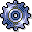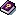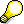Template-Application in Standard Cost EstimateUse

In contrast to the template-allocations (for cost objects, profitability segment, and others) the template-application is carried out implicitly during the normal cost calculation work steps, and not explicitly in its own transactions. Together with this, the template helps direct the overhead cost allocations from business processes or cost center/activity types to the costing object (see:Overhead costs).

You can apply the template in plan through:

• Costs calculation not generated by an order
• The system automatically determines the overhead costs in the cost estimate with quantity structure, when you carry out the calculation.
• The system determines the overhead costs in the cost estimate without quantity structure, in the additive calculation, and in the base planning and simulation calculation, once you save one of these calculation or if you choose the function Calculate overhead costs in the menu.
• Preliminary costing for a cost object
• Overhead costs are determined automatically during the preliminary costing of production orders with quantity structures, process orders and in the sales order costing (with the product costing method), when you carry out one of these calculations.
• Overhead costs are determined in the preliminary calculation of production orders without a quantity structure, in sales order costing (with the unit costing method) and in the general cost object planning, when you save one of these calculations or choose the function Calculate overhead costs in the menu.

Choose Template for Cost Objects and Cost Estimates in the standard cost estimate.

The template ascertains which overhead costs are used and to which extent. It also determines how these costs are assigned to the product. You can find the template through the costing sheet in the valuation variant (see: Assigning Templates for Cost Objects and Cost Estimates).You can assign overhead costs in actual through the template, while you execute a template-allocation in the period-end closing of cost object controlling (see: Template Allocation in Actual).